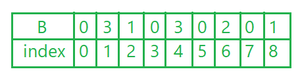Related Articles

# Java Program to Print All the Repeated Numbers with Frequency in an Array

• Difficulty Level : Easy
• Last Updated : 27 Nov, 2020

The frequency of an element in an array is the count of the occurrence of that particular element in the whole array. Given an array that may contain duplicates, print all repeated/duplicate elements and their frequencies.

Below is the discussion of this program by two approaches:

1. Using a counter array: By maintaining a separate array to maintain the count of each element.
2. Using HashMap: By updating the count of each array element in the HashMap<Integer, Integer>.

Example :

```Input :  arr[] = {1, 2, 2, 1, 1, 2, 5, 2}
Output : 1 3
2 4
// here we will not print 5 as it is not repeated

Input : arr[] = {1, 2, 3}
Output : NULL

// output will be NULL as no element is repeated.```

### Method 1:  (using counter array)

Explanation :

• The array can be sorted as well as unsorted.
• First, count all the numbers in the array by using another array.
• A be an array, A[ ] = {1, 6 ,4 ,6, 4, 8, 2, 4, 1, 1}
• B be a Counter array  B[x] = {0}, where x = max in array A “for above example 8”.
• In a for loop, initialized with i. Increase value in counter array for every element in array A.
• B [ A [i] ]++; this will give counter array asArray B after counting elements

• Finally print the index of B with its element whenever the element is greater than 1.

## Java

 `// Java program to count the frequency of``// elements in an array by using an extra``// counter array of size equal to the maximum``// element of an array`` ` `import` `java.io.*;`` ` `class` `GFG {``    ``public` `static` `void` `main(String[] args)``    ``{`` ` `        ``int` `A[] = { ``1``, ``6``, ``4``, ``6``, ``4``, ``8``, ``2``, ``4``, ``1``, ``1` `};`` ` `        ``int` `max = Integer.MIN_VALUE;``        ``for` `(``int` `i = ``0``; i < A.length; i++) {``            ``if` `(A[i] > max)``                ``max = A[i];``        ``}`` ` `        ``int` `B[] = ``new` `int``[max + ``1``];``        ``for` `(``int` `i = ``0``; i < A.length; i++) {`` ` `            ``// increment in array B for every integer``            ``// in A.``            ``B[A[i]]++;``        ``}``        ``for` `(``int` `i = ``0``; i <= max; i++) {``            ``// output only if element is more than``            ``// 1 time in array A.``            ``if` `(B[i] > ``1``)``                ``System.out.println(i + ``"-"` `+ B[i]);``        ``}``    ``}``}`

Output:

```1-3
4-3
6-2```

The above approach can consume more space which is equal to the maximum constraint of the element of array

Time Complexity: O(n)

Space Complexity: O(K) where K is the maximum element/constraint of an array

### Method 2: (Using HashMap)

• It is a simple method and has a base logic, you can do it by using an unordered Map as well.
• The map will save a lot of space and time.

## Java

 `// Java program to maintain``// the count of each element``// by using map.``import` `java.util.*;`` ` `class` `GFG {`` ` `    ``public` `static` `void` `main(String[] args)``    ``{`` ` `        ``int``[] a = { ``1``, ``6``, ``4``, ``6``, ``4``, ``8``, ``2``, ``4``, ``1``, ``1` `};``        ``int` `n = a.length; ``// size of array`` ` `        ``HashMap map = ``new` `HashMap<>();``        ``for` `(``int` `i = ``0``; i < n; i++) {`` ` `            ``if` `(map.containsKey(a[i])) {`` ` `                ``// if element is already in map``                ``// then increase the value of element at``                ``// index by 1`` ` `                ``int` `c = map.get(a[i]);``                ``map.replace(a[i], c + ``1``);``            ``}`` ` `            ``// if element is not in map than assign it by 1.``            ``else``                ``map.put(a[i], ``1``);``        ``}``        ``for` `(Map.Entry i :``             ``map.entrySet()) {`` ` `            ``// print only if count of element is greater``            ``// than 1.``            ``if` `(i.getValue() > ``1``)``                ``System.out.println(i.getKey() + ``" "``                                   ``+ i.getValue());`` ` `            ``else``                ``continue``;``        ``}``    ``}``}`
Output
```1 3
4 3
6 2```

Time Complexity: O(n)

Space Complexity: O(n)

Attention reader! Don’t stop learning now. Get hold of all the important Java Foundation and Collections concepts with the Fundamentals of Java and Java Collections Course at a student-friendly price and become industry ready. To complete your preparation from learning a language to DS Algo and many more,  please refer Complete Interview Preparation Course.

My Personal Notes arrow_drop_up